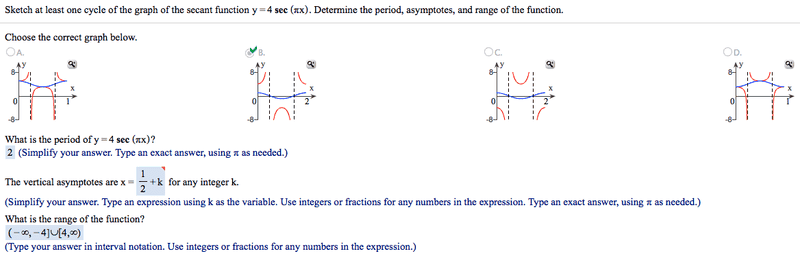# Trig: Writing the equation for vertical asymptote of a secant function?

## Homework Statement## Homework Equations

How did they come up with $\frac{1}{2}$+k for the equation of the vertical asymptote? I understand everything else except this.

## The Attempt at a Solution

On this particular exercise, I graphed it and saw that each of my vertical dashed lines were all one whole unit apart. I've tried this method with another problem that had the dashed lines separated 2 units apart, took that 2, and multiplied it by the x=$\prod$/2 + k$\prod$. The result was $\prod$+2k$\prod$, which was correct.

I tried it with other numbers and have gotten the correct answer, but I have a feeling I'm still doing something wrong. Because with this particular one using my method, I input $\prod$/2 + k$\prod$ as my answer for the vertical asymptote which was incorrect. What am I doing wrong?

Dick
I noticed that simply taking the 'B' (like in the y=Asin[B(x-C)]+D formula), turning it into the reciprocal (1/B), and thennnn multiplying it by $\prod$/2 + k$\prod$ gets me the right asymptote. This *does* relate to inverse trig functions (ie, sec being the reciprocal of cos), right?